# Effect of Layer Arrangement on 2-D Numerical Analysis of Freezing Process in Double Layer Porous Packed Bed

Effect of Layer Arrangement on 2-D Numerical Analysis of Freezing Process in Double Layer Porous Packed Bed
Center of Excellence in Electromagnetic Energy Utilization in Engineering (CEEE.) Department of Mechanical Engineering, Faculty of Engineering, Thammasat University (Rangsit Campus), Pathumthani 12120, Thailand

Department of Biological and Environmental Engineering, Cornell University, 208 Riley Robb Hall, Ithaca, NY 14853, USA

Corresponding Author Email:
Page:
273-284
|
DOI:
https://doi.org/10.18280/ijht.370133
7 January 2019
|
Accepted:
8 March 2019
|
Published:
31 March 2019
| Citation

OPEN ACCESS

Abstract:

The purpose of this study is to investigate the numerical model of heat and mass transfer during freezing process for various arrangements of porous layers within a rectangular enclosure. The numerical model for energy conservation in solid and liquid regions was solved by using the differential equations under the local thermal equilibrium condition (LTE). All governing equations with the entire computational domain including all regions were solved by the finite element method. A simulation model was established through the analysis based on two constraints: 1) the Boussinesq approach which considered in buoyancy term with the function of density inversion and 2) the local thermal condition. According to this model, the numerical predictions of velocity field in unfrozen zone reveal different flow behaviors resulting from the freezing load and layer arrangement. Some cases, such behaviors also depend on the operating time due to the increase of the frozen layer thickness which affect permeability. The velocity change in unfrozen zone also results in the moving rate and the shape of freezing front. Furthermore, it indicated that the characteristics of freezing process were dominated by freezing load and permeability in each layer. The 2-D model from this study reasonably correspond to the literatures.

Keywords:

freezing, solidification, double layers, layers arrangement, porous medium, permeability, freezing front, moving problem

1. Introduction

Solidification or freezing process in porous media are widely met in nature or in engineering systems, e.g. ice formation within the insulation, phase transformation of clued oil in pipe line, ice accretion on structures and machine, solidification of crystal or solidification from welding process, cryopreservation of food processing or engineering tissues. Especially, the solidification can be applied to waste treatment process which some contaminants can be extracted by using phase change technique. The systematical investigation on phase transition can be adopted to solve for many problems, i.e. food quality, foundation and structures damage from the frozen of groundwater. Furthermore, many researches have applied to analyze the industrial problems, especially in the cold regions.

Phenomena of heat and mass transfers in the porous media have been studied for many decades. As the first portion for thawing or melting process, Weaver and Viskanta  studied the melting of ice in porous media including the experiment and analysis for a cylindrical capsule with different sizes and types of spherical beads. The temperature distribution, shape and motion of solid- liquid interface were discussed. The analytical model included only conduction mode were compared with the experiment that was considered the significant of convective flow. Beckermann and Viskanta  reported the numerical and experimental study of phase change process in gallium and glass beads as fluid and porous media under the natural convection condition. Moreover, the transport equations were solved by the volume averaged. The predicted results showed the temperature and position of solid-liquid interface which reasonably agreed with the measurements. However, the convective heat transfer in the melting zone as well as the heat conduction in the solid were found that considerably influenced on the solid-liquid interface shape and the movement during phase change. Ng et al. considered the heat transfer during melting in a horizontal annulus with the finite element method under the latent heat thermal energy storage technique . It was employed to simulate the convection-dominated melting of a porous packed bed in enclosures. The effects of Rayleigh number on the melting rate as well as the evolution of the flow pattern which the increase in Rayleigh number promotes heat transfer rate were also studied. Chang and Yang studied the natural convection for the melting of ice in porous media in a rectangular enclosure by the numerical of SIMPLE Algorithm method with the local thermal equilibrium (LTE) condition , the influence of the maximum density parameter with the characteristic isothermal line, the influences of Darcy number and heat load at domain boundary with the fusion rate, and the shape of fusion interface were studied. Voller  applied the control volume finite element method (2-D) to analyze the modelling of unsaturated flow in soil layer, the saturation profiles in each layer, especially at the interface between layers were focused. Cheng and Lin analyzed the effects of melting on the transient mixed convective heat transfer from the vertical plate of saturated porous media . Rattanadecho and Wongwises presented the improvement of numerical model by combination of the transfinite interpolation technique and partial differential equation (PDE) method [7-8]. Firstly, preliminary grids were generated by transfinite interpolation with the algebraic method and the subsequent refinement by using a partial differential equation. The combination of two methods obtained the smooth grid generation, and accurately predicted the temperature distribution and the deformation shape of melting interface as well. Chaiyo and Rattanadecho  showed the numerical analysis of two dimensions for microwave melting of ice-saturated porous medium which was filled in a rectangular waveguide (TE10) at 2.45 GHz. Cheng et al.  used the enthalpy porosity technique based on the finite control volume method to solve the natural convection during phase change of binary mixture (carbon-iron), and the Navier-stokes equation was also applied by Boussinesq approximation technique. Furthermore, there are also interesting research groups including: Iwaki , Rihan and Abd El-Bary. .

The second portion for solidification or freezing process, Chellaiah and Viskanta presented the effects of liquid superheat and imposed temperature difference on freezing process within a rectangular of porous packed bed . The experimental and numerical methods which both conduction and natural convection were considered. According to the non-linear of density inversion of water, the freezing rate depended on the amount of superheat. The measured results compared with the numerical prediction presented a good agreement. Zhang and Hung Nguyen  studied the numerical investigation of the solidification for the superheated fluid‐porous medium which was contained in a rectangular cavity. The walls of the cavity are insulated except the top wall that was maintained at constant temperature and below the freezing point. The effects of superheat on the behaviors of the natural convection and the heat transfer during the process were concentrated. The front tracking method in a coordinate transform as a numerical technique was considered to simulate the moving boundary. Ismail and Henriquez  proposed the numerical study of the solidification of the phase change material in a spherical shell. The model was considered only a conduction mode within domain except the boundary conditions that were set under the constant temperature and convection heat transfer. The size of spherical shell, thickness of shell, material type, initial temperature of phase change material and the external wall condition affected the solidified mass fraction and the time to complete the solidification. Devireddy et al.  presented the numerical model with finite control volume for microscale heat and mass transport in biological tissue during freezing process. The coupled model was applied for two cryobiological freezing problems with different geometry and boundary conditions. The thermal properties of water and the biophysical properties of two biological tissues, normal rat liver and Dunning AT-1 rat prostate tumor tissue, were used to simulate both micro and macroscale freezing processes. Lu and Zhou  proposed the model of moving heat source and Green's function for solving the phase change problems that included freezing and thawing processes of biological skins. The temperature distributions, and the cooling and warming rates inside the skin subject under the two typical of boundary conditions which often encountered in skin cryo-preservation were analytically predicted. The effects of boundary conditions on the heat transfer performance approaches were comparatively discussed. Talamucci studied the freezing of liquid in underground and considered the flow of unfrozen liquid in underground that moved to freezing interface . Rattanadecho presented the experimental and numerical method for analyzing the freezing process of unsaturated granular packed bed with the LTE model . The rate of water absorption from unfrozen into the frozen layer depended on freezing heat flux and water saturation at solidification interface. Hasan and Louhi-Kultanen studied solidification of unsaturated water in Na2SO4(aq) which is a purification technique for waste water treatment . The influences of intensity, ambient temperature, freezing time, freezing load with efficiency of waste treatment were considered.

Mostly, the existing studies on the research problem that concerned the behaviors of heat and mass transfers including the phase changes inside porous materials were investigated under the single layer domain.  The analysis of the mass transfer behaviors clearly presented the consistency trends along the simulation time which differed from the such behaviors took place in the domain contained the packed bed of multi-layer porous materials. Therefore, this research attends to present the systematical investigation of the phase-change problems, i.e. the heat and mass transfers and the moving boundary of freezing interface for various arrangement of different porous layers. Moreover, the effects of temperature gradient between the boundary walls as well as the nonlinear density in terms of the pattern of natural convection in unfrozen zone which affect the shape of moving boundary are examined with transient model. The results can be applied in engineering design that concerned with phase change in any multiple layer porous materials, i.e. phase change in multi-layer of thermal insulation under various conditions. The study of ice propagation in multi-layer of underground soil to protect the underground structures, the cryogenic freezing for maintaining the quality of nutrients, i.e. fat, protein, sugar and so on, in food or any of bio-tissues.

2. Analysis of Heat Transfer and Freezing Interface

Figure 1 shows the two dimensions of physical model for freezing process of saturated water in porous media within a rectangular enclosure. Figure 1(a) shows single layer of porous pack bed which porosity is equal 0.4 while Figure 1(b) and 1(c) show different patterns for double layers arrangement of porous pack bed. Consideration for both type of double layers arrangement, it included for series left-right (LR) layer arrangement and parallel top-bottom (TB) layer arrangement. Differential porosity of porous layers has separated by vertical and horizontal arrangement. Namely, the porosity is equal 0.4 for left hand side of LR case and bottom layer of TB case and also equally 0.7 for right hand side of LR case and top layer of TB case. The porous media was full filled with phase change material (water). The layers of porous media in packed bed are glass bead and also there are two different sizes for double layers. Therefore, properties of both layers in packed bed for double layers cases are not equal, such as, porosity, permeability, effective thermal conductivity, effective thermal diffusivity, Prandtl number (Pr) and Darcy number (Da).

Initially, all domains have a uniform temperature and greater than the fusion temperature of a liquid (water). For time > 0, the increment of frozen zone is occurred from the left wall. Freezing is started at this wall, and interface between frozen and unfrozen zone moves from left to right according to Figure 2 as below.

## 1.pngFigure 1. Physical model

## 2.png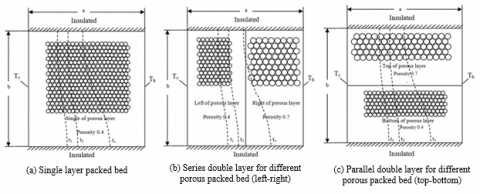Figure 2. Movements of freezing interface during freezing process

First of all, the basic phenomenon for freezing process in saturated porous media within a rectangular enclosure will be performed, the porous layer consists of two phase zones i.e., frozen zone and unfrozen zone. Inside the frozen layer, there is only heat conduction taking place, whereas inside the unfrozen zone, both of heat conduction and heat convection by natural convective heat transfer occurs. After time increase, consideration double layer case in Figure 2(b) when freezing interface moved through to the second layer, velocity and flow behavior of fluid in the unfrozen zone changed due to the variation of porosity ratio between double layers which have not yet freeze. It affects the behaviors of freezing interface such as shape and moving rate. Their behavior is changed compared the characteristic that are shown in the previous process. In addition, there is other pattern of double layer case as shown in Figure 2(c), it is also different behavior of freezing process which will be mentioned hereafter.

The assumptions for the analysis of freezing process in single layer, series double layer for different porous packed bed and parallel double layer for different porous packed bed are list as below:

1) The temperature field can be assumed to be two-dimensional.

2) The porous matrix and phase change material in pore are in the local thermal equilibrium condition (LTE).

3) The properties of solid matrix and phase change material are homogenous and isotropic.

4) The particles of solid matrix for each layer are considered to as spherical and uniform shape.

5) The porous matrix is saturated with phase change material (water in unfrozen layer and ice in frozen layer).

6) The fluid in pore is incompressible.

7) The frozen and unfrozen interface are clearly defined by fusion temperature Tf.

8) The properties of phase change material are all constant except the non-linear of density in the buoyancy term for liquid phase.

The density inversions of water between 0.01 and 12oC have been followed by a non-linear path, the maximum water density approximates of 4oC. The relationship between density and temperature has been formulated by Gebhart and Mollendorf  as:

ρ=ρm(1-w|T-Tm)(1)

where ρm is the maximum density (999.972 kg/m3) and $w=9.2793×10^(-6)(°C)^(-q)$, Tm=4.0293 ℃ and Q=1.894816, all constant are values proposed by Gebhart and Mollendorf. The Boussinesq method takes into account the effect of non-linear density variation in buoyancy term of the transport equations.

The differential of energy equation and momentum equation for two-dimensional heat transfers within both of frozen and unfrozen zone, respectively as:

Energy equation:

$(ρC_p )_{eff}\frac{∂T}{∂t}+(ρC_p )_{eff}(V∙∇T)=∇(k_{eff}∇T)$ (2)

The effective thermal properties of unfrozen and frozen zone can be expressed as below:

Unfrozen zone

$(ρC_p )_{eff}=(ερ_wC_{p,w})+(1-ε)(ρ_pC{p,p})$ (3)

$k_{eff}=εk_w+[1-ε]k_p$ (4)

Frozen zone

$(ρC_p )_{eff}=(ερ_i C_{p,i})+(1-ε)(ρ_pC{p,p})$ (5)

$k_{eff}=εk_i+[1-ε] k_p$ (6)

x -Momentum equation:

$\frac{1}{\varepsilon }\frac{\partial u}{\partial t}+\frac{u}{{{\varepsilon }^{2}}}+\frac{v}{{{\varepsilon }^{2}}}\frac{\partial u}{\partial y}=-\frac{1}{{{\varepsilon }^{2}}}\frac{\partial p}{\partial x}+\frac{\vartheta }{\varepsilon }(\frac{{{\partial }^{2}}u}{{{\partial }^{2}}x}+\frac{{{\partial }^{2}}u}{{{\partial }^{2}}y})-\frac{\mu u}{\rho fk}$ (7)

y-Momentum equation:

$\frac{1}{\varepsilon }\frac{\partial u}{\partial t}+\frac{u}{{{\varepsilon }^{2}}}\frac{\partial v}{\partial x}+\frac{v}{{{\varepsilon }^{2}}}\frac{\partial v}{\partial y}=-\frac{1}{\rho f}\frac{\partial p}{\partial y}+\frac{\vartheta }{\varepsilon }(\frac{{{\partial }^{2}}v}{\partial {{x}^{2}}}+\frac{{{\partial }^{2}}v}{\partial {{y}^{2}}})-\frac{\mu u}{\rho fk}+\rho g$ (8)

Continuity equation:

$\frac{\partial u}{\partial x}+\frac{\partial v}{\partial y}=0$ (9)

Initial condition:

$t=0 \,\,\,\, at\,\,\,\, 0≤x≤L, 0≤y≤H T=T_0$ (10)

$t=0\,\,\,\,at\,\,\,\,0≤x≤L, 0≤y≤H u=v=0$ (11)

Boundary condition:

$t>0\,\,\,\,at\,\,\,\,x=0, 0≤y≤H T=T_c$ (12)

$t>0\,\,\,\,at\,\,\,\,x=L, 0≤y≤H T=T_h$ (13)

$t>0\,\,\,\,at\,\,\,\,x=0,L 0≤y≤H u=v=0$ (14)

$t>0\,\,\,\,at\,\,\,\,y=0,H 0≤x≤H u=v=0$ (15)

$\frac{∂T}{∂y}=0\,\,\,\,at\,\,\,\,y=0,H 0≤x≤L$ (16)

3. Numerical Method

The transient problem of freezing process was solved by finite element method via the COMSOLTM Multiphysics. The physical domain was two dimensions and discretized by triangular element to approximate the temperature and velocity profile. For acceptable approximation, finer mesh was applied in the sensitive area such as at boundary domain. All governing equations were solved base on enthalpy porosity method and mesh was always fixed, therefore, the interpolation of temperature between meshes for fusion temperature could define the solidification interface or freezing front. Mesh convergence was found to be 15,000 elements which solution was independent. The result of mesh independent from the convergence test is shown in Figure 3 where the result showed relation between number of elements on x-axis and result of temperature at any point on y-axis. The initial time steps were varied during 0.01-1.0 second when the process started to be convergent. Moreover, the relative error in the iteration procedures of 10-6 was chosen.

## 3.png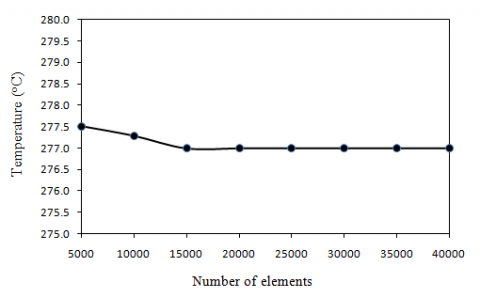Figure 3. Mesh independent analysis

4. Verification of the Model

In order to verification of the models, the solidification of pure metal at the vertical wall in the presence of liquid superheat by Wolff and Viskanta  were validated for comparing their rectangular geometric models with the numerical results from Comsol Multiphysics. In Figure 4 and 5, it shows that the results of solidification interface from Comsol Multiphysics are similar and good agreement with both of experimental and numerical results from Wolff and Viskanta . This favorable comparison lends confidence in the accuracy of the present numerical model.

## 4.pngFigure 4. Comparison of verification model predictions between present study (a), Wolff and Viskanta  (b)

## 5.pngFigure 5. Comparison of the calculated solidification interface position to the solidification interface position obtained by Wolff and Viskanta  for both of experimental data and prediction at time = 0.529 h

5. Result and Discussion

According to Figure.1, the physical model was set up as a rectangular enclosure with two dimensions of 6.5 cm × 6.5 cm which contained glass bead and was full filled with saturated water. Three case studies in Figures 1(a), 1(b) and 1(c), which were consisted of the single layer arrangement and double layer arrangement that included the series left-right (LR) layer arrangements case and parallel top-bottom (TB) layer arrangements case was considered. The temperature at the cold side (Tc) was maintained at -10 oC while the temperature at the hot side (Th) was varied between 4-10 oC. The results as shown in this section were about focused on the study of the natural convection inside the unfrozen zone, shape of solidification interface and freezing rate. The range of physical parameters are: Prandtl number (Pr) were 13.67 to 9.47, aspect ratio =1, Darcy number (Da)=3.74×10-4 to 1.07×10-2, the Raleigh number (Ra) were 6.39×105 to 3.29×106 and the Stefan number (Ste) were 0.05 to 0.13.

Figures 6–8 show the result of flow pattern in unfrozen zone by using the streamlines and vector flows for various time and conditions. As time increased, the region of frozen zone was expanded from left side while the volume of unfrozen zone was decreased. Consequently, those occurrences affected the natural convection inside. From Figures 9–11, show value of the streamlines in unfrozen zone for all cases study and the variations of freezing interface position by isothermal line at various times which conditions of layer arrangement and temperature at hot wall side were adjusted. The freezing interface moved from the cold side to the hot side whereas moving rate was depend on temperature at hot side and cod side. Furthermore, the orientation and arrangement of porous layers also affected to the freezing rate and distortion of freezing interface.

Figure 6 shows the stream line and vector flow of natural convection inside unfrozen zone for case of cold wall temperature (Tc) of -10oC and hot wall temperature (Th) of 10 oC. The area for right hand side and left hand side of domain are unfrozen and frozen zone. The characteristics of flow field, moving of freezing interface and shape of freezing interface at variation of time were predicted. Upper row, middle row and bottom row of Figure.6 are the phenomenon of freezing process for single layer case, series left-right (LR) layer arrangements case and parallel top-bottom (TB) layer arrangements case, respectively. The behaviors of natural convection inside unfrozen zone for all patterns of layer arrangement are almost identical. The direction flow of bigger current is counter-clockwise, which is unlike that of the smaller current. These patterns take place only for phase transition of water inside domain with the maximum density of water at 4 oC.

## 6.pngFigure 6. Behaviors of freezing process and natural convection inside unfrozen layer for three cases study of layer arrangement on time dependent with Tc = -10oC and Th = 10oC

Furthermore, Figure 6 shows two convective currents within the unfrozen zone, counter-clockwise and clockwise directions for large and small convective currents. The distortions of freezing interface in Figure 6 resulted from the energy exchange at upper portion of freezing interface, then gradually decreased when fluid move through to the lower portion. So, the freezing rate of lower portion was faster than the upper portion. In case of the unfrozen zone near the upper portion of freezing interface with time less than 14,400 s. in Figure 9 was considered, the fluid velocity in TB case dominate both the LR and the single layer case, respectively. This is because of the porosity at upper portion of the TB case was the highest influence when compared with single layer case and LR case, as well as the permeability in the TB case was the highest also. In addition, the velocity of natural convection at the top of domain is always higher than the bottom for counter clockwise flow which hot wall was set at the right-hand side. Whereas time was greater than 14,400 s, the fluid velocity of the LR case was the greatest and single layer case was the slowest. According to these results, it was because of the circulated current at the left side of the porous material for LR case was changed into the ice with its porosity of 0.4 while the circulated current as water was remained at the right side of the porous material with the porosity of 0.7. Consequently, the fluid velocity in pores was faster and also affects the freezing rate.

## 7.pngFigure 7. Behaviors of freezing process and natural convection inside unfrozen layer for three cases study of layer arrangement on time dependent with Tc=-10oC and Th=7oC

Figure 7 shows the stream line and vector flow of natural convection inside unfrozen zone for case of Tc of -10oC and Th of 7oC. The characteristics are dissimilar when compared with Figure 6, especially in case of the behavior of natural convection inside unfrozen zone and shape of freezing interface resulted from the variation of time.

According to the results at Th of 7oC in Figure 7, it was found that there were also the smaller and bigger currents of natural convection inside unfrozen zone. The convection of smaller currents expanded to nearly the upper portion of freezing interface and also affected shape variation of their interface. In additionally, the curve of the freezing interface was gradually change form curve line to be vertical straight line. For considering the main current of natural convection in unfrozen zone as shown in Figure 10 at time less than 7,200 s, the velocity was the highest for TB case and was slowest for single layer case. Nevertheless, for time higher than 7,200 s, the velocity for LR case was the highest instead the TB case and single layer case was also slowest. In accordance with this phenomenon, the reason was similar as in Figure.6 but it took place faster than that at time of 7,200 s because of hot temperature at right wall was changed to be 7oC. Furthermore, since the small currents in Figure 7 was bigger than that in Figure 6 at the same time. Therefore, the decline of heat transfer from the right wall exactly affected the behaviors of freezing interface both of shape and freezing rate when compared with Figure 6.

For all cases of the arrangement layer in Figure 8, the hot wall temperature (Th) at the right side of domain was changed to be 4oC that cause the water density was the highest. This situation resulted in to take place the only main current of the circulated water in the unfrozen zone.

As Figure.8 shows the stream line and vector flow of natural convection inside unfrozen zone which Tc of -10oC and Th of 4oC. Since the flow behavior as natural convection at Th of 4oC resulted from the maximum density, the main current was the clockwise in the unfrozen zone and that was different with Figures 6 and 7. Especially, the shape of freezing interface at lower portion was slower than the upper portion. The overall consideration for velocity of natural convection flow in unfrozen layer according to Figure 11, the fluid velocity at lower portion of domain of the LR case was the highest while the fluid velocity of the single layer case was the slowest. Therefore, the freezing rate of LR case was the slowest and that of the single layer case was the fastest.

## 8.png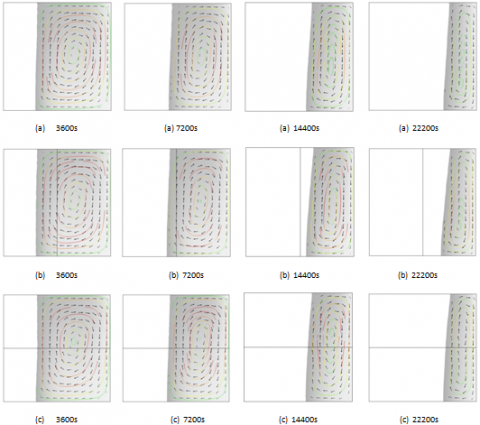Figure 8. Behaviors of freezing process and natural convection inside unfrozen layer for three cases study of layer arrangement on time dependent with Th=4oC

## 9a.png## 9b.png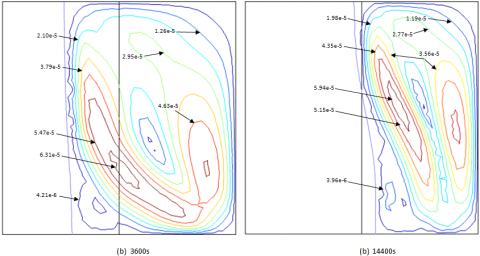## 9c.pngFigure 9. Contour variations of velocity at various of time for Th=10oC (Ra=1.71×106 to 3.29×106, Ste=0.11, Da=3.74×10-4 to 1.07×10-2)

## 10a.png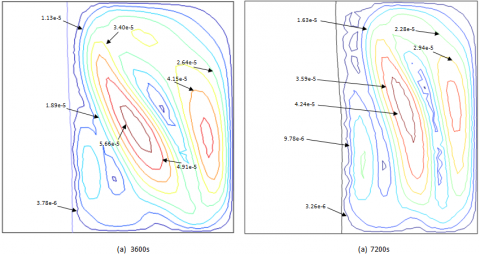## 10b.png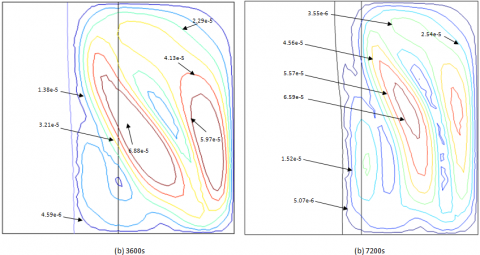## 10c.pngFigure 10. Contour variations of velocity at various of time for Th=7oC (Ra=1.16×106 to 2.24×106, Ste=0.11, Da=3.74×10-4 to 1.07×10-2)

## 11a.png## 11b.png## 11c.pngFigure 11. Contour variations of velocity at various of time for Th=4oC (Ra=6.39×105 to 1.24×106, Ste=0.11, Da=3.74×10-4 to 1.07×10-2)

Figure 12, the freezing interface which Tc of -10oC and Th of 10oC at different position and times for all cases were considered. It was found that the freezing rate was fast during the initial process, then was slow with time increase. This resulted from the thickness of ice layer that grew up as the thermal insulation. Initially, the ice growth of freezing interface at upper portion for TB case was the slowest compared with LR case and single layer case, that was because the fluid velocity as mentioned in Figures.6 and 9.

While the freezing rate of LR case at t>14,400 s became to the slowest since only the layer at the right side of domain was still remained the circulated current as water with 0.7 of porosity while the circulated current at the left side of the porous material was changed into the ice with its porosity of 0.4. On the other hand, the unfrozen layer of TB case, in which the fluid circulated, was separated to the half-upper portion with 0.7 of porosity and half-below portion with 0.4 of porosity.

## 12.png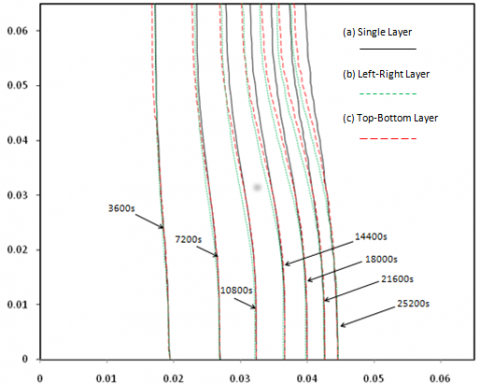Figure 12. Variations of the interface positions at various values of time for Th=10oC

## 13.png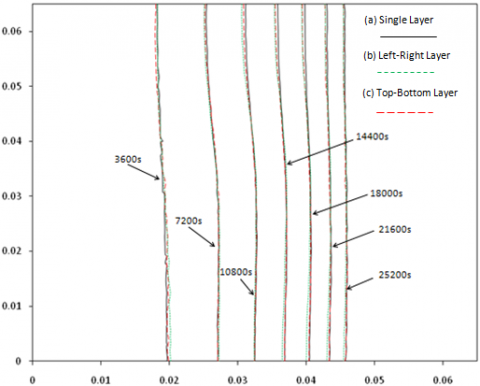Figure 13. Variations of the interface positions at various values of time for Th=7oC

Figure.13 shows the freezing interface which Tc of -10oC and Th of 7oC at different position and time for all cases were considered. The freezing rate at Th of 7oC are faster than that at Th of 10oC and the distortion of freezing interface also decreased due to the Th change from 10oC to 7oC. After the initial process, the freezing rate still decreased with time increase since the insulation of ice-layer growth.

According to Figure.13 with Th=7oC, it was noticed that at t=3,600 s the freezing rate of TB case was the slowest compared with single layer and LR cases which were almost similar freezing rate. Whereas, considering the freezing rate at t≥7,200 s, it was the slowest for LR cases but was the fastest for single layer case. These behaviors are similar as the study at Th=10oC except less time consumption. In addition, the freezing rates of LR case and TB case were not quite different resulting from the unusual extension of the smaller current at Th=7oC was bigger than that at Th=10oC.

## 14.png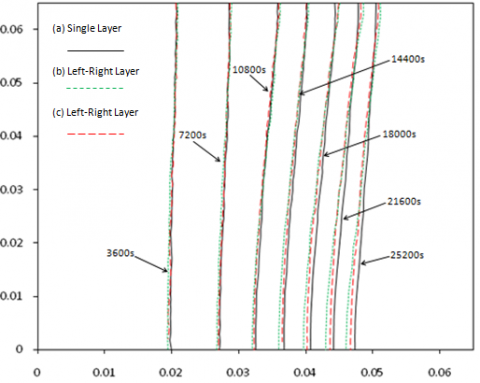Figure 14. Variations of the interface positions at various values of time for Th=4oC

In case of the examination at Th=4oC, the position changes of freezing interface of porous layer for all pattern arrangement were shown in Figure 14. For all three cases, the freezing rate at the upper portion of freezing interface was faster than that at bottom portion since the initial process. It was caused the clockwise main-current in the unfrozen zone. Then, the freezing rate of the freezing interface at the end of the process gradually slows which is the effect of thermal resistance. This was indicated that the freezing rate of the LR case was the lowest while the freezing rate of single layer was highest. This phenomenon is effect from porosity arrangement that makes highest permeability for LR case and lowest permeability for single layer case.

6. Conclusions

Summarizing the result of numerical analysis, it has for some conclusions;

(1) The relationship between non-linear of density variation with temperature has affect with flow field-pattern and direction of flow, temperature distribution, freezing rate and shape of freezing interface.

(2) Consideration temperature different between hot and cold side, when the difference become larger, the isothermal line of freezing interface distorts more, main eddy current is bigger but inverse for smaller eddy current.

(3) The results were occurred only main eddy current as clockwise direction when Th equal 4 oC. The lower part of freezing interface bends to the left-hand side more than the upper part.

(4) When porosity or permeability increase, the heat transfer also better, greater distortion of freezing interface but lower of freezing rate.

(5) The behavior of freezing process, such as, natural convection as an eddy current flow inside unfrozen zone, freezing rate and shape of freezing interface are depend on time and layers arrangements.

(6) The freezing rated gets poor when porosity or permeability increase, it’s also greater distortion of freezing interface.

Based on the results obtained, the extension work for phase change phenomena with complicated assumptions are considered, i.e. phase changes based on the Local Thermal non-Equilibrium (LTNE) condition., The phenomena of heat and mass transfer in mixed fluid during phase transition.

Acknowledgment

The authors gratefully acknowledge the financial support for this work provided by The Thailand Research Fund (TRF) under the Royal Golden Jubilee Ph.D. Program (RGJ) contract no. PHD/0017/2555, the Thailand Research Fund (TRF) contract No. RTA5980009 and the Thailand Government Budget Grant.

Nomenclature
 asf specific surface area of porous matrix [m2] Cp heat capacity [J/kg×K] Da Darcy number [-] dp particle diameter [m] g gravity [m/s2] h heat transfer coefficient [W/m2×K] hsf heat transfer coefficient between phase change material in pore and surface of porous matrix [W/m2×K] k thermal conductivity [W/m×K] kf thermal conductivity of fluid [W/m×K] kw thermal conductivity of water [W/m×K] ks thermal conductivity of solid particle [W/m×K] ki thermal conductivity of ice [W/m×K] P Pressure [N/m2] Pr Prandtl number [-] Ra Raleigh number [-] Ste Stefan number [J/kg×m] T temperature [oC] Tw temperature of water [oC] Ti temperature of ice [oC] T∞ ambient temperature [oC] Tm maximum density temperature = 4.029325 °C t time [s] u x-direction velocity [m/s] v y-direction velocity [m/s] w 9.2793*10-6 (oC)-q x x-coordinate [m] y y-coordinate [m] Greek symbols ρ density [kg/m3] ρm maximum density [kg/m3] ρv water density [kg/m3] ρf fluid density [kg/m3] ε porosity [-] v kinematic viscosity [m2/s] μ dynamic viscosity [N×s/m2] μ thermal expansion coefficient [1/K] Subscripts q 1.894816 Subscripts eff effective parameter
References

 Weaver JA, Viskanta R. (1986). Melting of frozen porous media contained in a horizontal or a vertical cylindrical capsule. International Journal of Heat and Mass Transfer 29(12): 1943-1951. http://dx.doi.org/10.1016/0017-9310(86)90013-X

 Beckermann C, Viskanta R. (1988). Natural convection solid/liquid phase change in porous media. International Journal of Heat and Mass Transfer 31(1): 35-46. http://dx.doi.org/10.1016/0017-9310(88)90220-7

 Ng KW, Gong ZX, Mujumdar A. (1998). Heat transfer in free convection-dominated melting of a phase change material in a horizontal annulus. International Communications in Heat and Mass Transfer 25: 631-640. http://dx.doi.org/10.1016/S0735-1933(98)00050-5

 Chen WJ, Yang DF. (1996). Natural convection for the melting of ice in porous media in a rectangular enclosure. International Journal of Heat and Mass Transfer 39(11): 2333-2348. http://dx.doi.org/10.1016/0017-9310(95)00310-X

 Voller VR. (2002). A control volume finite element solution of unsaturated flow in layered soils. Developments in Water Science 47: 105-112. http://dx.doi.org/10.1016/S0167-5648(02)80051-X

 Cheng WT, Lin CH. (2006). Transient mixed convective heat transfer with melting effect from the vertical plate in a liquid saturated porous medium. International Journal of Engineering Science 44: 1023-1036. http://dx.doi.org/10.1016/j.ijengsci.2006.05.008

 Rattanadecho P, Wongwises S. (2007). Simulation of freezing of water-saturated porous media in a rectangular cavity under multiple heat sources with different temperature using a combined transfinite interpolation and PDE methods. Computers and Chemical Engineering 31: 318-333. http://dx.doi.org/10.1016/j.compchemeng.2006.07.016

 Rattanadecho P. (2008). Moving boundary-moving mesh analysis of freezing process in water-saturated porous media using a combined transfinite interpolation and PDE mapping methods. ASME Journal of Heat Transfer 130: 1-10. http://dx.doi.org/10.1115/1.2780177

 Chaiyo K, Rattanadecho P. (2013). Numerical analysis of heat-mass transport and pressure buildup of unsaturated porous medium in a rectangular waveguide subjected to a combined microwave and vacuum system. International Journal of Heat and Mass Transfer 65: 826-844. http://dx.doi.org/10.1016/j.ijheatmasstransfer.2013.06.066

 Cheng WT, Huang EN, Chuo MH, Du SW. (2012). Transient natural convective heat transfer in porous medium with solidification of binary mixture. International Communications in Heat and Mass Transfer 39: 1132-1137. http://dx.doi.org/10.1016/j.icheatmasstransfer.2012.06.007

 Iwaki T. (2000). Molecular dynamics study of lattice defect generation during very rapid solidification. International Journal of Heat and Technology 18(1): 43-48. http://dx.doi.org/10.18280/ijht.18sp0106

 Rihan Y, Abd El-Bary B. (2010). Numerical study on the effect of solidification parameters during the continuous casting of Al-Si alloys. International Journal of Heat and Technology 28(2): 13-17. http://dx.doi.org/10.18280/ijht.280203

 Chellaiah C, Viskanta R. (1989). Freezing of water-saturated porous media in the presence of natural convection: Experiments and analysis. ASME Journal of Heat Transfer 111(2): 425-432. http://dx.doi.org/10.1115/1.3250694

 Zhang X, Hung Nguyen T. (1999). Solidification of a superheated fluid in a porous medium effect of convection. International Journal of Numerical Methods for Heat and Fluid 9(1): 72-91. http://dx.doi.org/10.1108/09615539910251112

 Ismail KAR, Henríquez JR. (2000). Solidification of PCM inside a spherical capsule. Energy Conversion and Management 41: 173-187. http://dx.doi.org/10.1016/S0196-8904(99)00101-6

 Devireddy RV, Smith DJ, Bischof JC. (2002). Effect of microscale mass transport and phase change on numerical prediction of freezing in biological tissues. ASME Journal of Heat Transfer 124: 365-374. http://dx.doi.org/10.1115/1.1445134

 Lu J, Zhou YX. (2002). Analytical study on the freezing and thawing processes of biological skin with finite thickness. Heat and Mass Transfer 38: 319-326. http://dx.doi.org/10.1007/s002310100216

 Talamucci F. (2003). Freezing processes in porous media: formation of ice lenses, swelling of the soil. Mathematical and Computer Modelling 37: 595-602. http://dx.doi.org/10.1016/S0895-7177(03)00053-0

 Rattanadecho P. (2004). Experimental and numerical study of solidification process in unsaturated granular packed bed. Journal of Thermophysics and Heat Transfer 18(1): 87-93. http://dx.doi.org/10.2514/1.9155

 Hasan M, Louhi-Kultanen M. (2015). Ice growth kinetics modeling of air-cooled layer crystallization from sodium sulfate solutions. Chemical Engineering Science 133: 44-53. http://dx.doi.org/10.1016/j.ces.2015.01.050

 Gebhart B, Mollendorf JC. (1977). A new density relation for pure and saline water. Deep Sea Research 24: 831-848. http://dx.doi.org/10.1016/0146-6291(77)90475-1

 Wolff F, Viskanta R. (1988). Solidification of a pure metal at a vertical wall in the presence of liquid superheat. International Journal of Heat and Mass Transfer 31(8): 1735-1744. http://dx.doi.org/10.1016/0017-9310(88)90285-2